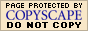Basic "Percent of" Word Problems (page 1 of 3)

Sections: Basic percentage exercises, Markup / markdown, General increase / decreaseWhen you learned how to translate simple English statements into mathematical expressions, you learned that "of" can indicate "times". This frequently comes up when using percentages.

If you need to find 16% of 1400, you first convert the percentage "16%" to its decimal form; namely, the number "0.16". (When you are doing actual math, you need to use actual numbers. Always convert the percentages to decimals!) Then, since "sixteen percent OF fourteen hundred" tells you to multiply the 0.16 and the 1400, you get: (0.16)(1400) = 224. This says that 224 is sixteen percent of 1400.

Percentage problems usually work off of some version of the sentence "(this) is (some percentage) of (that)", which translates to "(this) = (some decimal) × (that)". You will be given two of the values, or at least enough information that you can figure two of them out. Then you'll need to pick a variable for the value you don't have, write an equation, and solve for that variable.

• What percent of 20 is 30?

We have the original number (20) and the comparative number (30). The unknown in this problem is the rate or percentage. Since the statement is "(thirty) is (some percentage) of (twenty)", then the variable stands for the percentage, and the equation is:

30 = (x)(20)

30 ÷ 20 = x = 1.5

Since x stands for a percentage, I need to remember to convert this decimal back into a percentage:

1.5 = 150%

Thirty is 150% of 20.

• What is 35% of 80?

Here we have the rate (35%) and the original number (80); the unknown is the comparative number which constitutes 35% of 80. Since the exercise statement is "(some number) is (thirty-five percent) of (eighty)", then the variable stands for a number and the equation is:

x = (0.35)(80)

x = 28

Twenty-eight is 35% of 80.

• 45% of what is 9?

Here we have the rate (45%) and the comparative number (9); the unknown is the original number that 9 is 45% of. The statement is "(nine) is (forty-five percent) of (some number)", so the variable stands for a number, and the equation is:

9 = (0.45)(x)

9 ÷ 0.45 = x = 20

Nine is 45% of 20.

The format displayed above, "(this number) is (some percent) of (that number)", always holds true for percents. In any given problem, you plug your known values into this equation, and then you solve for whatever is left.

• Suppose you bought something that was priced at \$6.95, and the total bill was \$7.61. What is the sales tax rate in this city? (Round answer to one decimal place.)

The sales tax is a certain percentage of the price, so I first have to figure what the actual tax was. The tax was:

7.61 – 6.95 = 0.66

Then (the sales tax) is (some percentage) of (the price), or, in mathematical terms:

0.66 = (x)(6.95)

Solving for x, I get:

0.66 ÷ 6.95 = x = 0.094964028... = 9.4964028...%

The sales tax rate is 9.5%.

In the above example, I first had to figure out what the actual tax was. Many percentage problems are really "two-part-ers" like this: they involve some kind of increase or decrease relative to some original value. Warning: Always figure the percentage of change relative to the original value.

• Suppose a certain item used to sell for seventy-five cents a pound, you see that it's been marked up to eighty-one cents a pound. What is the percent increase?

81 – 75 = 6

The price has gone up six cents. Now I can find the percentage increase over the original price.

Note this language, "increase/decrease over the original", and use it to your advantage: it will remind you to put the increase or decrease over the original value, and then divide.

This percentage increase is the relative change:

6/75 = 0.08

...or an 8% increase in price per pound.

Top  |  1 | 2 | 3  |  Return to Index  Next >>

 Cite this article as: Stapel, Elizabeth. "Basic 'Percent of' Word Problems." Purplemath. Available from     https://www.purplemath.com/modules/percntof.htm. Accessed [Date] [Month] 2016

MathHelp.com Courses
This lesson may be printed out for your personal use.WyzAnt Tutoring